## Matrix Rank

Recalled in the previous topics when we have a set of basis vectors , we can concatenate these vectors into a matrix. Using this matrix, we can also change the basis or the coordinate system. In this page, you will learn more about that augmented matrix.

Suppose we have a matrix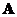size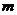by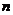. We can imagine this matrix is actually a vertical concatenation of row vectors. A set of row vectors that form a matrix is called row space of the matrix.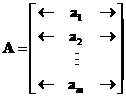Similarly, we can imagine the matrixis actually a horizontal concatenation of column vectors. A set of column vectors that form a matrix is called column space of the matrix.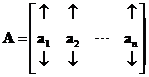The dimension of row space of a matrix is equal to the dimension of the column space of that matrix. The common dimension of the row space and column space of matrixis called the rank of matrix, denoted by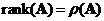. The rank ofis the number of linearly independent vectors and equal to the dimension of space spanned by those vectors. In other words, rank ofis the number of basis vectors we can form from matrix.

Performing elementary row operation does not change the row space. To compute the rank of a matrix, we reduce the matrix into reduced row echelon form (RREF) and non-zero rows of the RREF matrix will form the basis for the row space.

The interactive program below produces matrix rank. The computation is based on numerical method of Singular Value Decomposition (SVD). The input is rectangular matrix. Random Example button will provide you with many examples.

## Properties

Some important properties of matrix rank are:

• Transpose operation does not change the rank of a matrix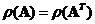.
• Multiplication of a matrix with its transpose does not change the rank of the matrix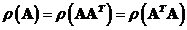.
• Rank of a matrix sizebyis always less or equal to the minimum size of the matrix. A matrix is said to be of full rank when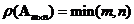.
• Let matrixand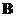both are matrices of the same sizeby, not necessarily of full rank, then we have the following
o Rank of matrix addition :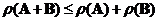o Rank of matrix subtraction :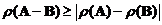• Let matrix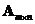and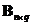are not necessarily the same size, then we have the following
o Rank of matrix product :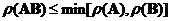o Rank of matrix product compare to summation of rank: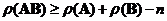• The dimension of the range and the null space of a matrix are related through fundamental relationship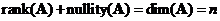.
• A linear system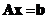has a solution if and only if the rank of the coefficient matrix is equal to the rank of the augmented matrix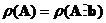. When non-homogeneous system is not full rank or when the rank of the matrix coefficients is less than the rank of the augmented matrix coefficients and the vector constants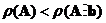then the system is usually inconsistent with no possible solution. It is still possible to find the approximate solution using generalized inverse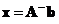.
• A matrixhas a left inverse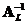if and only if its rank equals its number of columns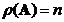. If matrixhas more columns than rows (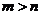), it cannot have a left inverse.
• A matrixhas a right inverse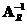if and only if its rank equals its number of rows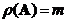. If matrixhas more rows than columns (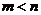), it cannot have a right inverse.
• A matrixhas an inverse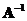( non-singular ) if and only if it is a square matrix and the rank of the matrix is full, that is the rank equals to the number of rows (or columns)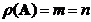. If matrixis not square (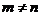), it cannot have a two-sided inverse. Equivalently, the determinant of the matrix is non-zero.